# Test: Money- 1

## 10 Questions MCQ Test Mathematics for Class 1 | Test: Money- 1

Description
Attempt Test: Money- 1 | 10 questions in 15 minutes | Mock test for Class 1 preparation | Free important questions MCQ to study Mathematics for Class 1 for Class 1 Exam | Download free PDF with solutions
QUESTION: 1

### Which of the following pouch has more money?

Solution:

Pouch 1 has 15 rupees. Pouch 2 has 19 rupees. Pouch 3 has 21 rupees. Pouch 4 has 18 rupees.

QUESTION: 2

### Which of the following you can buy from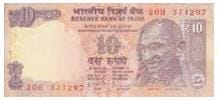note?

Solution:

Pencil which is of Rs. 5 can be bought from Rs. 10 note.

QUESTION: 3

### Which set of coins shows Rs. 5?

Solution:

50p+50p = Rs.1. Rs.1+Rs.1+ Rs.2+Rs.1 = Rs.5

QUESTION: 4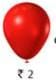can be taken from?

Solution:

Four 50p coins makes Rs. 2.

QUESTION: 5

Mohan has four Rs. 5 coins, out of which he gave 3 Rs. 5 coins to buy a toy. How much money, he is left with?

Solution:

4 Rs. 5 coins, =? 20. 3 coins of Rs. 5 = Rs. 15. 3 coins =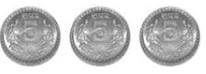He is left with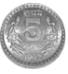QUESTION: 6

Money is counted in terms of notes and _________ .

Solution:

Money is counted in terms of notes and coins.

QUESTION: 7

Which of the following note does not exist?

Solution:

15 rupees note does not exist.

QUESTION: 8

Identify the note given in the picture and choose the correct option?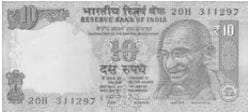Solution:

It is a 10 rupee note.

QUESTION: 9

Which of the following will you use to buy a toy car of Rs. 50?

Solution:
QUESTION: 10

Which note you will use to pay for the given item of worth Rs. 9?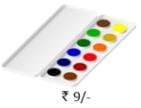Solution:

A 10 rupee note will be used to water colours worth Rs. 9 to purchase.Use Code STAYHOME200 and get INR 200 additional OFF Use Coupon Code